×

×

Subjects / Electrical Engineering / Electrical Engineering / EE 114 / in materials such as metals, the outer shell electrons are loosely bou

# in materials such as metals, the outer shell electrons are loosely bou Description

##### Description: THESE NOTE COVERS WHAT WOULD BE IN OUR NEXT EXAM. ITS ELECTRICAL ENGINEERING SCIENCE 1
55 Pages 194 Views 1 Unlocks
Reviews

UNESCO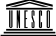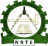UNESCO-NIGERIA TECHNICAL &  VOCATIONAL EDUCATION  VOCATIONAL EDUCATION  REVITALISATION PROJECT REVITALISATION PROJECT-PHASE II  NATIONAL NATIONAL DIPLOMA IN  ELECTRICAL ENGINEERI ELECTRICAL ENGINEERING TECHNOLOGY NG TECHNOLOGY Low High Low High _I+ _V+ 100 volts volts 10 Ohms R ELECTRICAL ENGINEERING  ELECTRICAL ENGINEERING  ELECTRICAL ENGINEERING SCIENCE (I) SCIENCE (I) COURSE CODE COURSE CODE: EEC 115  YEAR I YEAR I- SEMESTER I THEORY  Version 1: December 2008 Version 1: December 2008 TABLE OF CONTENTS ELECTRICAL ENGINEERING SCIENCE (I) THEORY  LIST OF CONTENTS WEEK 1: 1.1 Atom 1.2 Structure and composition of an atom 1.3 Conductors, insulators and semiconductor  WEEK 2: 1.4 Concepts of current and electron flow 1.5 Electric current, potential difference, electromotive force and resistance 1.6 Multiples and sub multiples of electric quantities WEEK 3:  2.1 Direct current  2.2 Analogy between current flow and water flow  2.3 Basic d.c. circuit WEEK 4  2.4 Ohm’s law WEEK 5:  2.4 Ohm’s law WEEK 6 :  2.5 Resistivity and conductivity  2.6 Series and parallel circuit WEEK 7:  2.7 Solved problems on resistivity and conductivity 2.8 Equivalent resistance of series and parallel circuits WEEK8:  2.9 Kirchhoff’s laws WEEK 9:   2.10 Superposition principle WEEK 10:  2.11 Temperature coefficient of resistance WEEK 11:   3.1 Energy and its various types  3.2 Relationship between electrical, mechanical and thermal energy  3.3 Electrical power WEEK 12:  3.4 Joule’s law WEEK 13:  4.1 Electric charge  4.2 Coulomb’s law of electrostatics WEEK 14:  4.3 Definition of terms used in electrostatics  4.4 Capacitance WEEK 15:  4.5 Capacitor connections  4.6 Energy stored in a capacitorConcept Of Electric Current Flow Week 1 At the end of this week, the students should be able to:  • Define an atom  • Explain the structure and composition of an atom  • Differentiate between conductors, insulators and semi conductors  1.1 ATOM  Defination: An atom is the smallest indivisible particle which all matter is made of up.  1.2 STRUCTURE AND COMPOSITION OF AN ATOM  According to the electron theory of matter, an atom is made up of tiny particles. The central  part of the atom is known as the nucleus. The nucleus of the atom consists of particles called  protons and neutrons held together in a compact form by a binding energy. The outer portion  of the atom is made up of orbiting particles called electrons. Electrons normally move about  the centre of an atom in paths which are referred to as shells orbits. Each of these shells can  contain only a certain maximum number of electrons. The central part of the atom shows its  nucleus. We should note that protons are positively charged particles while electrons are  negatively charged particles, since opposite charges attract, the negatively charged electrons  are attracted to the positively charged protons in the nucleus. Consequently, this electric force  of attraction holds the electrons in their orbit.  The number of orbiting electrons normally equals the number of protons in the nucleus. For  that reason an atom is said to be neutrally charged.  1.3 CONDUCTORS, INSULATORS AND SEMICONDUCTOR  Conductor In a conductor, electric current can flow freely, in an insulator it cannot. Metals such as  copper typify conductors, while most non-metallic solids are said to be good insulators,  having extremely high resistance to the flow of charge through them. "Conductor" implies  that the outer electrons of the atoms are loosely bound and free to move through the material.  Most atoms hold on to their electrons tightly and are insulators. In copper, the valence  electrons are essentially free and strongly repel each other. Any external influence which 5 moves one of them will cause a repulsion of other electrons which propagates, "domino  fashion" through the conductor.  Types of conductors and their properties  Many types of materials can conduct electricity.  Take these materials listed here and put them in order from lightest to heaviest  with respect to their densities, that is, weight per unit volume:    aluminum, copper, gold, iron, lead, mercury, silver, water, zinc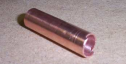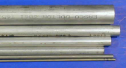aluminum copper Insulator An Insulator is a material or object which resists the flow of heat (thermal insulators) or  electric charge (electrical insulators).  The term insulator has the same meaning as the term dielectric, but the two terms are  used in different contexts. The opposite of insulators are conductors and semiconductors,  which permit the flow of charge. Semiconductors are strictly speaking also insulators, since  they prevent the flow of electric charge at low temperatures, unless doped with atoms that  release extra charges to carry the current). However, some materials (such as silicon dioxide)  are very nearly perfect electrical insulators, which allow flash memory technology. A much  larger class of materials, (for example rubber and many plastics) are "good enough"  insulators to be used for home and office wiring (into the hundreds of volts) without  noticeable loss of safety or efficiency.  6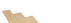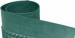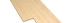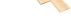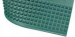wood  Rubber  Semiconductor  A semiconductor is a material with an  is a material with an conductance that is intermediate between that of an  that is intermediate between that of an  insulator and a conductor.  A semiconductor behaves as an insulator at very low temperature, and  A semiconductor behaves as an insulator at very low temperature, and  A semiconductor behaves as an insulator at very low temperature, and has an  appreciable conductance at room temperature room temperature. A semiconductor can be distinguished from a  inguished from a  conductor by the fact that, at absolute zero absolute zero, the uppermost filled electron energy band is fully  electron energy band is fully  filled in a semiconductor, but only partially filled in a conducto filled in a semiconductor, but only partially filled in a conductor. The distinction between a  r. The distinction between a  semiconductor and an insulator is slightly more arbitrary. A semiconductor has a  semiconductor and an insulator is slightly more arbitrary. A semiconductor has a  semiconductor and an insulator is slightly more arbitrary. A semiconductor has a band gap which is small enough such that its  which is small enough such that its conduction band is appreciably thermally populated with  thermally populated with  electrons at room temperature, whilst an insulator has a band gap which is too wide for there  electrons at room temperature, whilst an insulator has a band gap which is too wide for there  electrons at room temperature, whilst an insulator has a band gap which is too wide for there to be appreciable thermal electrons in its conduction band at to be appreciable thermal electrons in its conduction band at room temperature. Band structure of a semiconductor Band structure of a semiconductor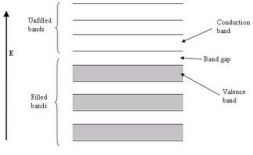Fig 1.1: Band structure of a semiconductor showing a full valence band and an empty  conduction band. The Fermi level lies within the forbidden band gap  In the parlance of solid-state physics, semiconductors (and insulators) are defined as  solids in which at absolute zero (0 K), the uppermost band of occupied electron energy states,  known as the valence band, is completely full. Or, to put it another way, the Fermi energy of  the electrons lies within the forbidden band gap. The Fermi energy, or Fermi level can be  thought of as the energy up to which available electron states are occupied at absolute zero.  At room temperatures, there is some smearing of the energy distribution of the  electrons, such that a small, but not insignificant number have enough energy to cross the  energy band gap into the conduction band. These electrons which have enough energy to be  in the conduction band have broken free of the covalent bonds between neighbouring atoms  in the solid, and are free to move around, and hence conduct charge. The covalent bonds from  which these excited electrons have come now have missing electrons, or holes which are free  to move around as well. (The holes themselves don't actually move, but a neighbouring  electron can move to fill the hole, leaving a hole at the place it has just come from, and in this  way the holes appear to move.)  It is an important distinction between conductors and semiconductors that, in  semiconductors, movement of charge (current) is facilitated by both electrons and holes.  Contrast this to a conductor where the Fermi level lies within the conduction band, such that  the band is only half filled with electrons. In this case, only a small amount of energy is  needed for the electrons to find other unoccupied states to move into, and hence for current to  flow.  The ease with which electrons in a semiconductor can be excited from the valence  band to the conduction band depends on the band gap between the bands, and it is the size of  this energy bandgap that serves as an arbitrary dividing line between semiconductors and  insulators. Materials with a bandgap energy of less than about 3 electron volts are generally  considered semiconductors, while those with a greater bandgap energy are considered  insulators..  The current-carrying electrons in the conduction band are known as "free electrons,"  although they are often simply called "electrons" if context allows this usage to be clear. The  holes in the valence band behave very much like positively-charged counterparts of electrons,  and they are usually treated as if they are real charged particles. 8 Concept Of Electric Current Flow Week 2 At the end of this week, the students should be able to:  • Explain the concepts of current and electron flow  • Define electric current, potential difference, electromotive force (e.m.f) and resistance  • State multiples and submultiples of electric quantities  1.4 CONCEPT OF CURRENT AND ELECTRON FLOW  At any instant in time, the electrons in a conductor are in random motion. However, if a  directional force e.g. electromotive force EMF from a battery is applied to the conductor as  shown in Fig 2.1 below, then end A of the conductor is positive while end B is negative. This  results in directed flow of electrons.  The directional movement of free electrons is referred to as current flow and the conventional  current flow is in the opposite direction of electron flow as can be seen in the diagram.  Normally, conventional current flow is generally used.    Power  source  B  A  -ve terminal  Electron flow  Current flow +ve terminal   Fig 2.1  1.5 ELECTRIC CURRENT, POTENTIAL DIFFERENCE, ELECTRO-  MOTIVE FORCE AND RESISTANCE  Electric Current Electric current is the rate of flow of electric charges round a circuit. It has a symbol I and is  measured in amperes (A).  Potential Difference (P.d.)  The potential difference between two points is defined as the number of joules of electrical  energy transferred from one side of the points to the other with the passage of one coulomb  from one point to another. It is measured in volts (V).  9 Electromotive Force (e.m.f) Electromotive force is the force that gives rise to electric current in a circuit; also it’s the  force that makes flow of electric current in a circuit. This force arises from many effects  including chemical (e.g. battery cells) and magnetic, as (e.g. generator). The unit of  electromotive force is the volt, symbol E.  Resistance  The resistance can be defined as an opposing force experience by the flow of charge through  a material. The opposition is due to the collision between electrons and other atoms in the  material, and it converts electrical energy into heat energy. The unit of resistance is Ohm (Ω),  and has a symbol R.  1.6 MULTIPLES AND SUBMULTIPLES  Since it is often necessary to describe quantities that exist in large multiples or submultiples  of a unit, therefore standard prefixes are used to denote powers of 10 of SI units. See below  table;  Table 2.1. SI prefixes PREFIX SYMBOL MULTIPLIER Tera T 1012 Giga G 109 Mega M 106 Kilo K 103 Hector h 102 Deka da 101 Deci d 10-1 Centi c 10-2 Milli m 10-3 Micro µ 10-6 Nano n 10-9 Pico p 10-12

## 1 KΩ and the voltage to 50V, what is the new value of current?Don't forget about the age old question of 1319015883

10 Simple d.c. Circuits Week 3 At the end of this week, the students should be able to:  • Define d.c current  • State the analogy between current flow and water flow  • Describe basic d.c. circuits  2.1 DIRECT CURRENT  Direct current is that current which flows in only direction at a time. The d.c. circuits  normally contain a battery, which is the source, and this battery has two terminals namely:  Negative and Positive terminals as shown in figure 3.1 below.   + -   Fig. 3.1: symbol of a battery  2.2 ANALOGY BETWEEN CURRENT FLOW AND WATER FLOW  The three main concepts in electricity are voltage, current and resistance. Voltage or potential  difference can be thought of as the driving force (although it is not really a "force") behind  the electric current. In the water analogy, in figure (3.2), you can think of voltage as the  pressure difference created by a pump that causes the water to flow through the pipe in the  water system. Also you can think of the electrons flow in the electric circuit as equivalent to  the flow of the water in the pipes that caused by the pump.  Another important concept in electricity is resistance. It’s Property of a material that  opposes the flow of electrons through it. There is always a resistance flow between two  points in a pipe. This is analogous to the resistance (R) in an electric circuit. 11 Figure (3.2) water analogy 2.3 BASIC D. C. CIRCUIT  A basic d.c. (direct current) circuit consist of an electrical connection of a d.c power source  (e.m.f source), connecting wires and a load as shown in Fig 3.3. In this case, the load can be  any electrical component (e.g. a resistor) or a network of resistors. The d.c. power sources  such as dry cells (such as used in flash lights), car batteries and laboratory d.c power supply  unit. The symbol of a d.c source is as shown in Fig. 3.3. It is represented by two vertical  lines, with the longer line marked (+) and the shorter line marked (-).  E.m.f,  source    E + _ R (load)  Connecting wire  Fig 3.3  12 Concept Of Electric Current Flow Week 4 At the end of this week, the students should be able to:  • State ohm’s law  • Explain ohm’s law  • Draw the graph of current versus voltage  • Draw the graph of current versus resistance  2.4 OHM’S LAW In 1826 George Simon Ohm found that the current, voltage, and resistance are  related in a specific way. Ohm expressed this relationship with a formula that is  known today as Ohm‘s law. In this chapter, you will learn Ohm‘s law and how to used  it in solving circuit problems.  Electric circuit can be of two basic forms: series and parallel. In this chapter,  series, and parallel circuits are studied. you will also see how Ohm‘s law is used in  series, and parallel circuits   Ohm‘s law is the most important mathematical relationship between  voltage, current and resistance in electricity. Ohm’s Law is used in three forms  depending on which quantity voltage, current or resistance you need to  determine. In this section, you will learn each of these forms.  2.4.1 Explanation of Ohm‘s Law  In the electric circuit, if the voltage across constant resistor value is increase,  the current through the resistor will also increase; and, if the voltage is decrease, the  current will decrease. For example, if voltage is double, the current will doubled. If  the voltage is halved, the current will also be halved. This relationship is illustrated in  the figure (4.1), with used voltage and current meter. 13 Low High Low High + 10 Ohms 200 volts _I R _V + (a) Decrease V, decrease I (b ) Increase V, increase I  Figure (4.1): Effect of changing the voltage at constant resistance  Ohm‘s law also stated that if the voltage is kept constant, less resistance results  in more current, and, also, more resistance results in less current. For example, if  resistance is halved, the current will doubled. If the resistance is doubled, the current  is halved.  This relationship is illustrated by the meter indications in the figure (4.2).  Where the resistance is increase and the voltage is constant.  (a) Decrease R, increase I (b ) Increase R, decrease I  Figure (4.2): Effect of changing the resistance at constant voltage   From previous illustrated,  Ohm‘s law states that, current and voltage are linearly proportional at constant resistance; current and resistance are  inversely related at constant voltage. According this law the following three equivalent formula is derived:  14 Formula for current  I = V R   Equation (4.1)  This form of Ohm‘s low is used to determine current if voltage and resistance values  are known  Formula for voltage  V = I R   Equation (4.2)  This form of Ohm‘s low is used to determine voltage if current and resistance values  are known  Formula for resistance R == V I  Equation (4.3)  This form of Ohm‘s low is used to determine resistance if voltage and current values  are known  2.4.2 Ohm‘s Law triangle  There is an easy way to remember which formula to use. By arranging current,  voltage and resistance in a triangle, one can quickly determine the correct formula.  Ohm‘s Law triangle is shown in figure (4.3)   Figure(4.3) : Ohm‘s Law triangle  15 To use the triangle, cover the value you want to calculate, the remaining letters make  up the formula as shown in figure (4.4).  V = I R R == V I I = V R  Figure(4.4) : Easy way to remember which formula to use  Remember the following three rules: Ohm‘s Law can only give the correct answer when the correct values are used.  Current is always expressed in Amperes or Amps (A)  Voltage is always expressed in Volts (V)  Resistance is always expressed in Ohms (Ω)  2.4.3The Relationship of Current, Voltage and Resistance  Ohm‘s law describes how current is related to voltage and resistance. current  and voltage are linearly proportional at constant resistance; current and resistance are  inversely related at constant voltage.  2.4.3.1 The Linear Relationship of Current and Voltage  Current and voltage linearly proportional; that is, if one is increased by a  certain percentage, the other will increase or decrease by the same percentage,  assuming that the resistance is constant value.  To draw a graph of current verses voltage, let‘s take a constant value of  resistance. For example, R=10Ω, and calculate the current for several value of voltage  ranged from 0 to 100 V by used the formula I=V/10 where R=10 Ω. the current values  obtained are shown in the table (4.1):  16   V(v) I(A) 10 1  20 2  30 3  40 4  50 5  60 6  70 7  I = V  10 ΩI (A) 7 6 5 4 3 2 1 0

= 10 Ω

R

## 1 KΩ and the voltage to 50V, what is the new value of current?Don't forget about the age old question of psychology 201 exam 1

Table (4.1)   0 10 20 30 40 50 60 70 Figure (4.5) : Graph of current versus voltage for   R= 10 Ω  The graph of the current values versus the voltage values is shown in the Fig.  (4.5). This graph tells us that a change in voltage results in a linearly proportional  change in current. From graph the change in voltage from 20 to 30 is increased by  50% by calculation the current must be increase by the same percentages 50% I = 2 +  2 X 50/100 = 3A.  2.4.3.2 Current and Resistance are Inversely Related   Current varies inversely with resistance as expressed ohms law, I=V/R. when  the resistance is decreased, the current goes up; when the resistance is increased, the  current goes down. For example, let‘s take a constant value of voltage V=10volts, and  calculate the current for several value of resistance ranged from 10 to 100 Ω by used  the formula I=10/R. the current values obtained are shown in the next table (3-2),  The graph of the current values versus the voltage values is shown in the figure(3-6).  17 R (Ω) I (A)  10 1  20 0.500 30 0.333 40 0.250 50 0.200 60 0.167 70 0.143 I (A) 0.6 0.5 0.4 0.3 0.2 0.1

## In the circuit in figure (3-9), how much resistance is needed to draw 4mA of current ?We also discuss several other topics like uab si

I = 10 v  R Table ( 4.2)  0 R (Ω) 0 10 20 30 40 50 60 70 0Figure (4.6) : Graph of current versus resistance for  V= 10 v 18 Simple d.c. Circuits Week 5  At the end of this week, the students should be able to:  • Calculate current using ohm’s law  • Calculate voltage using ohm’s law  • Calculate the resistance using ohm’s law  2.4.4 Calculating Current  In this section, you will learn to determine the current values when you known  the values of voltage and resistance. As examples, by using the following formula I =  V/R . In order to get current in amperes, you must express the value of voltage in volt  and the value of resistance in ohms.  Example 5.1   The voltage supplied by the battery is 10 volts, and the resistance is 5 Ω  in the circuit of figure (3-7). Calculate the current in amperes?  IV  10v Figure (6.1) Solution: use the formula  R  10Ω V = 100 volts      I = V  R R = 10 Ω I = ? 10   I = = 1 A  10    19 Example5-2   If the resistance in figure 5.7 is changed to 0.1 KΩ and the voltage to   50V, what is the new value of current?  Solution:  V  I = R     V = 500 volts  R = 0.1 KΩ 50   I = = 0.25 A  100  2.4.5 Calculating Current   = 0.1 X 1000 Ω    = 100 Ω I = ?    In this section, you will learn to determine the current values when you known the  values of voltage and resistance. As examples, by using the following formula I =  V/R . In order to get current in amperes, you must express the value of voltage in volt  and the value of resistance in ohms.  Example 5.1   The voltage supplied by the battery is 10 volts, and the resistance is 5 Ω  in the circuit of figure (3-7). Calculate the current in amperes?  Figure (5.2) Solution: use the formula  V = 100 volts  I =   V  R = 10 Ω R I = ? 10   I = = 1 A    10 20 Example 5.2   If the resistance in figure 3-7 is changed to 0.1 KΩ and the voltage to   50V, what is the new value of current?  Solution:  I =   V  V  R 10v I R  V = 500 volts  10Ω R = 0.1 KΩ 50   I = = 0.25 A  100   = 0.1 X 1000 Ω    = 100 Ω I = V  I = ?    2.4.6 Calculating Resistance  R In this section, you will learn to determine the resistance values when you  known the values of voltage and current. As examples, by using the following formula  R = V/I   In order to get resistance by ohms, you must express the value of voltage in  volts and the value of current in amperes.    Example 5.5   In the circuit in figure (3-9), how much resistance is needed to draw 4mA of  current ?  I = 6A V  24 v Figure (5.3) Solution:   use the formula R= V / I  R = V I  R  V = 24 v  R = ?  I = 6A      24   R = = 4 Ω   6 21 Example 5.6   The circuit in figure (5.3), the voltage is changed to 1.2 KΩ, and the current  is changed to 4 mA, what is the resistance  Solution:   use the formula R= V / I    V = 1.2 kV   = 1.2 X 1000   = 1200V  V  1200   R = = = 3,000,000 Ω R = ?  I 4×1000  I = 4 mA   If required Resistance by M Ω  R = 3,000,000 /1,000,000 = 3 MΩ      22 Simple d.c. Circuits Week 6  At the end of this week, the students should be able to:  • Define resistivity and conductivity of a conductor  • State the relationship between series and parallel circuits  • Differentiate between series and parallel circuits  2.5 RESISTIVITY AND CONDUCTIVITY  Resistivity  Resistivity ρ of a material is the resistance of unit length of material and unit cross sectional  area at a given temperature.   At a given temperature, the resistance R, of a given material is:  • directly proportional to the length of the material, l, and  • inversely proportional to the cross sectional area of the material, A.  mathematically, these two statements can be combined and stated as   R = α l   (5.1)  A  where ρ is a constant of proportionality known as resistivity of the material, (ρ) is Greek  letter pronounced Rho.)   from equation (5.1), we can deduce that   ρ = R X A Ohm X meter2 = Ohm-meter   l meter   We should note that when R is in ohms, A in m2 and l in m, then the unit of ρ is ohm meter (Ω - m)  Conductivity  The inverse of resistivity is called conductivity and is denoted by the symbol σ. (σ is a Greek  letter pronounced sigma) its unit of measurement is (Ω - m)-1, or 1 .   Ω - m  Mathematically, the two terms can be related as   σ = 1 , (Ω - m)-1   (5.2)   ρ This relationship can be expressed as follows:  the higher the resistivity of a material, the lower its conductivity, and vice versa. 23 2.6 SERIES AND PARALLEL CIRCUITS  Series circuits  A series circuit is formed when any numbers of resistors are connected end-to start so that there is only one path for current to flow. The resistors can be actual  resistors or other devices that have resistance like lamps.  Figure (6.1) shows two resistors connected in series (end -to –start) between  points A and B. There is only way for current to get from point A to point B. a series  circuit provides only one path for current between two points in a circuit so that the  current in the same through R1and R2.  Figure (6.1) : Resistor in series  In an actual circuits diagram, a series circuit may be easy to identify as those in  figure (6.1) For example. Figure (6.2) shows series resistors drawn in other ways.   Figure (6.2) : Some examples of series circuits. Notice that the current is the   same at all points Parallel Circuits  A parallel circuit is formed when any number of resistors are connected starts-to-starts  and ends-to-ends so that there is different paths for current to flow. The resistors can be  actual resistors or other devices that have resistance like lamps. 24 Figure (6.3) shows two resistors connected in parallel (start-to-start and end-to-end)  between points A and B. There is two ways for current to get from point A to point B. a  parallel circuit provides more than one path for current between two points in a circuit so that  the current flow in two paths current flow in R1 is called I1 and current flow in R2 is called I2.  Figure (6.3) : Resistor in series  In an actual circuits diagram, a parallel circuit may be easy to identify as those in  figure (6.3). For example. Figure (6.4) shows Some examples of parallel resistors drawn in  other ways.  Figure (6.4) : Some examples of parallel circuits. Notice that the current is   the same at all points25 Simple d.c. Circuits Week 7  At the end of this week, the students should be able to:  • Solve problems involving resistivity and conductivity  • Deduce the equivalent resistance of series and parallel circuits  • Solve problems on series and parallel resistors  2.7 SOLVE PROBLEMS INVOLVING RESISTIVITY AND  CONDUCTIVITY  Example 7.1  A cylinder wire of 0.5m in length, 0.5mm in diameter, has a resistance of 2.5Ohms. calculate  the  resistivity of the wire.  Solution  length, l = 0.5m   radius r = 0.5mm = 0.25 mm = 2.5 X 10-4m   2   Cross sectional area, A = πr2 = 22 X (2.5 X 10-4)2 m2  7  ρ = RA = 2.5 X (22/7) X (2.5 X 10-4)2  l 0.5  ∴ ρ = 98.19 X 10-8 Ohm – meter.  Example 7.2  A wire of diameter 0.6mm, resistivity 1.1 X 10-6Ω - m has resistance of 44Ω. Calculate the  length of  the wire.  Solution  Here, we are given:   radius, r = 0.6 mm = 3 X 10-4m   2  To find l we deduce it from ρ = RA (i.e. l = RA )   l ρ ∴ l = 44 X (22/7) X (3 X 10-4)2  1.1 X 10-6  = 11.3m 26 Example 7.3  A 30m conductor of cross sectional area 2mm2 has a resistance of 10Ω. Calculate the  conductivity  of the conductor.  Solution  ρ = l .  RA   = 30 = 1.5 X 106 (Ω - m)-1  10 X 2(10-3)2 2.8 EQUIVALENT RESISTANCE OF SERIES AND PARALLEL CIRCUIT  Equivalent Resistance Of A series Circuit  I1 R1I2 R2 R3 I3V(R1) V(R2) V(R3)  I  Vs  Figure 7.1 Series circuit  When resistors of resistance R1, R2 and R3 are joint together (fig 7.1), they are said to  be connected in series. It should be noted that:  (i) The current through a series circuit is the same all over.  (ii) The voltage drop across each resistor is different depending on its resistances.  (iii) The applied voltage (Vs) is equal to the sum of the P.d’s across each resistor.  i.e. I=I1=I2=I3  Vs= V(R1) + V(R2) +V(R3)   (7.1)  where Vs = IR; V(R1)= IR1; V(R2) = IR2; V(R3) = IR3   (7.2) Putting eqtn (7.2) in (7.1) gives   IR = IR1 + IR2 + IR3    => Req = R1 + R2 +R3  For n resistors connected in series, the equivalent resistance  27  Req. is given by Req. = R1 + R2 +R3 + …. + Rn   (7.3)  Equivalent Resistance Of A Parallel Circuit  I  Vs   I1 R1 I2 R2 I3 R3Figure 7.2 Parallel circuit  When resistors of resistance R1, R2 and R3 are connected in parallel, the following  statements hold good:  (i) The p.d across each resistor is the same  (ii) The current flowing through each resistor is different  (iii) The supply current (I) is equal to the sum of current flowing through each  resistor  i.e. Vs = V1 = V2 = V3   I = I1 + I2 + I3   (7.4)  where I = Vs/R; I1 = Vs/R1 ; I2 = Vs/R2; I3 = Vs/R3   (7.5) Inserting eqtn (7.5) in (7.4) gives   Vs/R = Vs/R1 + Vs/R2 + Vs/R3 ⇒ 1/R = 1/Req = 1/R1 + 1/R2 + 1/R3  For n resistors connected in parallel,  1/Req = 1/R1 + 1/R2 + 1/R3 + - - - + 1/Rn   (7.6)    28 Example 7.4   In the circuit in figure (7.3), Determine the two voltages drops across R1 and   R2?  + Figure (7.3) Solution:  R1= 2.2 Ω  1) First solve for total resistance.   RT = R1 + R2   = 2.2 + 1.8 = 4 Ω  2) Second, solve for current:  R2= 1.8 Ω V = 12 v  I = ?  VR1= ?    V  12   I = = = 3 Amp  VR2= ?RT 4   3) Finally, solve for voltage drop across any resistor:  Voltage drop across R1  VR1= I X R1 = 3 X 2.2 = 6.6 v  Voltage drop across R2  VR2= I X R2 = 3 X 1.8 = 5.4 v  The sum of all voltage drops ( 6.6v + 5.4v ) equals the source voltage (12v).  29 Example 7.5   In the circuit in figure (7.4), Determine the current in each branch  + Figure (7.4) Solution:  1) First solve for total resistance.  Used the formula when there are two unequal value resistors  R1 X R2 4 X 6  24   RT = = = = 2.4 Ω   R1 + R2 4 + 6  10   2) Second, solve for voltage across any resistor:     VR1 = VR1 = VT = 12 v   3) Finally, solve for current in each branch :  R1= 4 Ω R2= 6 Ω   VR1 12  V = 12 v   I1 = = = 3 Amp    R1 VR1 4  12  I1 = ?   I2 = = = 2 Amp  I2 = ?    R1 VT 6  12   IT = = = 5 Amp  RT 2.4 The sum of current in each branch (3 + 2) equal the total current 5A    30 Simple d.c. Circuits Week 8  At the end of this week, the students should be able to:  • State Kirchhoff’’s laws  • Explain Kirchhoff’s laws  • Solve simple problems on Kirchhoff’s laws  2.10 KIRCHHOFF’S LAWS  Kirchhoff’s laws were developed in 1847 by the German physicist G.R. Kirchhoff. These  laws are two in numbers and they are formally known as Kirchhoff’s current law (KCL) and  Kirchhoff’s voltage law (KVL).  Kirchhoff’s current law  Kirchhoff’s current law state that the algebraic sum of currents entering a node is zero.   By this law for purposes of explanation, we may regard currents entering a node as  positive, where currents leaving the node as negative. Considering the node in Fig 8.1 and  applying KCL, we get   i1 + (-i2) + (-i3) + i4 + i5 = 0   (8.1)  or i1 + i4 + i5 = i2 + i3   (8.2)  From equation (8.2) we notice that the sum of current entering a node is equal to the sum of  the current leaving the node. This is an alternative way of stating KCL.  i5  i4  Fig 8.1  i1  i2  i3 31 Example 8.1  Find the value of I in Fig 8.2  Solution  6A 4A P 8A I 5A Fig 8.2   In order to find the value of I, the following equation can be formed.   Currents towards P = currents flowing from P.  ∴ 6 + 4 + 5 = I + 8, ⇒ I = 7A  Kirchhoff’s voltage law  Kirchhoff’s voltage law state that in a closed circuit, the algebraic sum of the e.m.f.s is equal  to the algebraic sum of the voltage drops.   Mathematically this can be stated as:  ± E1 + ±E2 = ± I1R1 ± I2R2 ± I3R3,   if there are two sources of e.m.f and three resistors R1, R2 and R3 In general, ∑ E = ∑ IR  Example 8.2  Determine the value of Vab in the circuit shown in Fig 8.3 (a) and (b) using KVL.  6V + a  6V + a  Vab 3V  _ b  2V  _ +  Vab 3V  _ b  2V  (a) (b)  Fig 8.3  32 Solution  For Fig 8.3(a), applying KVL, we get   -2V + 3V – 6V – Vab = 0  ∴ Vab = -5V   For Fig 8.3 (b) applying KVL, we get   -2V + 3V + 6V – Vab = 0  ∴ Vab = 7V  Example 8.3  Applying KCL and KVL to the circuit of Fig. 8.4, write down the equation pertaining to loop  I and II respectively,  R1 I1 I2 I3 R3 I II R2 E1 E2  Fig 8.4  Solution  N.B. we are working under the assumption of fixed direction of I1, I2 and I3 as shown  in Fig 8.4   Applying KVL to loop I, we get   -E1 = R1I1 + R2I2  Applying KVL to loop II, we get   E2 = -R2I2 + R3I3  From KCL we known that at node A   I1 = I2 + I3  or I3 = I1 – I2  Putting this value of I3 into the equation involving E2, we get   E2 = -R2I2 + R3 (I1 – I2)   E2 = R2I2 – (R2 + R3) I2 33 Simple d.c. Circuits Week 9  At the end of this week, the students should be able to:  • State superposition principle  • Explain superposition principle  • Solve simple problems on superposition principle  2.11 SUPERPOSITION PRINCIPLE  The superposition principle is a method that allows us to determine the current  through or the voltage across any resistor or branch in a network. The advantage of  using this approach instead of Kirchhoff’s laws is that it is not necessary to use  determinant or matrix algebra to analyze a given circuit. The theorem state the  following:  In a circuit containing several sources of e.m.f., the resultant current in any  branch is the algebraic sum of the currents in that branch that would be produced by  each e.m.f, acting alone, all other sources of e.m.f being replaced meanwhile by their  respective interval resistances.  In order to apply the superposition principle it is necessary to remove all  sources other than the one being examined. In order to ‘’Zero’’ a voltage source, we  replace it with a short circuits, since the voltage across a short circuit is zero volts. A  current source is zeroed by replacing it with an open circuit, since the current  through an open circuit is zero amps.  Example 8.1  Solve the same problem in example 3.5 to determine all the branch currents using the  superposition principles.  Solution   To solve this problems we shall follow the steps.  Step I, Re-draw the given circuit diagram with E1 = 15V and E2 = OV, as shown in  Fig 9.1 below 34 I1 A I3 I2 I1” I2’ A  I3” 4Ω 2Ω 3Ω E1 = 15V E2 10V  B  4Ω E1 = 15V  3Ω 2Ω B   (a) Original circuit diagram (b) circuit diagram with E2 =OV  Fig 9.1  Step II: Next, determine all the branch currents I1’, I2’ and I3’ indicated in Fig 9.1 (b),   With E1 = 15V and E2 = OV.  Step III: we find the equivalent resistance of 3Ω resistor and 2Ω resistor connected in  parallel.   i.e. R3Ω//R2Ω = 3 X 2 = 6 = 1.2Ω  3 + 2 5  Step IV: The circuit diagram of Fig 9.1(b) reduces to the form shown in Fig 9.1  4Ω R3Ω//R2Ω = 1.2ΩE1 = 15V   Fig 9.2  Step V: we can apply the Kirchhoff’s Voltage law to the circuit diagram of Fig 9.2 to obtain,   I’1 = E1 .  (4 + R3Ω//R2Ω)   I’1 = 15 = 2.885A   ( 4 + 1.2)  Step VI: Turn back to fig 9.1(b) and bear in mind that I1 is the total current that flows into  the 3Ω resistor branch and 2Ω resistor branch which are in parallel. Applying the principle of  current division rule, we get   I’2 = R2Ω X I’1 = 2 X 2.885 = 1.154A   (R2Ω) + R3Ω) (2 + 3)  35 Similarly, I’3 = R3Ω X I’1 = 3 X 2.885 = 1.731A   (R2Ω + R3Ω) (2 + 3)  Step VII: Draw the corresponding circuit diagram if the original circuit diagram of Fig 9.1  (a) has E1 = OV and E2 = 10V, to produce the circuit diagram shown in Fig. 9.3(b)  I1 A I3 I2 I1” A  I3” I2’ 4Ω 2Ω 3Ω E1 = 15V E2 10V  B  4Ω 3Ω B  E2 = 10V  2Ω (a) Original circuit diagram (b) circuit diagram with E1 = OV   Fig 9.3  Following all the stages in step II above, we obtain the following results:  (i) R4Ω//R3Ω = 4 X 3 = 12 Ω = 1.714Ω  4 + 3 7  (ii) The resulting equivalent circuit diagram after combing R4Ω and R3Ω in parallel gives  Fig 9.4.  2ΩR4Ω//R3Ω E2 = 10V   Fig 9.4  (iii) Applying Ohm’s law to the circuit diagram of Fig 9.4, we get   I”3 = E2 = 10 ≅ 2.693A   2 + R4sΩ // R3Ω (2 + 1.714)  (iv) with reference to Fig 9.3, we see that if I”3 is to serve as the total current then  according to KCL then I”1 and I”2 must be seen to flow into node A. For  (v) this to happen the direction I”2 must be opposite to what is indicated in the diagram of  Fig 9.3. This means that I”2 must bear a negative value. Only I”1 flows into node A.  Therefore, I”1 = 3 X 2.693 = 1.154A   (4 + 3)  36 Similarly, I”2 = _ 4 X 2.693 = - 1.539A   (4 + 3)  Step VIII: The total branch currents can be obtained by adding up algebraic as follows:   I1 = I”1 + I’1 = 2.885 + 1.154 = 4.039A   I2 = I’2 + I”2 = 1.154A – 1.538A = - 0.384A   I3 = I’3 + I”3 = 1.731A + 2.693A = 4.424A 37 Simple d.c. Circuits Week  10  At the end of this week, the students should be able to:  • Define temperature coefficient of resistance  • Draw the graph of resistance against temperature  • Solve simple problems on temperature coefficient of resistance  2.12 TEMPERATURE COEFFICIENT OF RESISTANCE  If the resistance of a coil of insulated copper wire is measured at various temperatures up to  say 2000C, it is found to vary as shown in the figure 10.1 below.  Resistance (Ω)   1.426

Don't forget about the age old question of Why would soil and water be added to a compost pile?